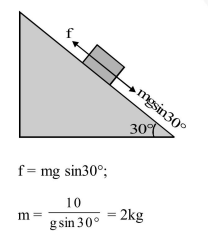Deepak Scored 45->99%ile with Bounce Back Crack Course. You can do it too!

# A block rests on a rough inclined plane making an angle of

Question:

A block rests on a rough inclined plane making an angle of $30^{\circ}$ with the horizontal. The coefficient of static friction between the block and the plane is $0.8$. If the frictional force on the block is $10 \mathrm{~N}$, the mass of the block (in kg) is : (taken $\mathrm{g}=10 \mathrm{~m} / \mathrm{s}^{2}$ )

1. $2.0$

2. $4.0$

3. $1.6$

4. $2.5$

Correct Option: 1

Solution: